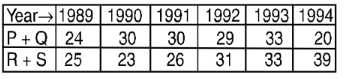## Introduction to Data Interpretation

#### Data Interpretation

Direction: Following table gives the population of a locality from 1988 to 1992. Read the table and answer the questions.1. The number of children in 1988 is :

1. According to given table, we have
Total population in 1988 = 146947
Total number of men and women in 1988 = 65104 + 60387 = 125491
The number of children in 1988 = Total population in 1988 - Total number of men and women in 1988

##### Correct Option: D

According to given table, we have
Total population in 1988 = 146947
Total number of men and women in 1988 = 65104 + 60387 = 125491
The number of children in 1988 = Total population in 1988 - Total number of men and women in 1988
The number of children in 1988 = 146947 – 125491 = 21456

Direction: The table given here shows production of five types of cars by a company in the year 1989 to 1994. Study the table and answer questions.1. The percent increased in total production of all types of cars in 1992 to that in 1991 was :

1. As per the given above table , we can see
Total production of all types of cars in 1992 = 90
Total production of all types of cars in 1991 = 75
Increase in production = 90 - 75 = 15

 Required answer = Increase in production × 100 =% Total production of all types of cars in 1991

##### Correct Option: B

As per the given above table , we can see
Total production of all types of cars in 1992 = 90
Total production of all types of cars in 1991 = 75
Increase in production = 90 - 75 = 15

 Required answer = Increase in production × 100 =% Total production of all types of cars in 1991

 Required answer = 15 × 100 = 20% 75

1. The production of which type of car was 25% of the total production of all types of cars during 1993?

1. On the basis of given table in question ,
Total production of all types of cars during 1993 = 80
According to question,

 25% of 80 = 25 × 80 100

##### Correct Option: A

On the basis of given table in question ,
Total production of all types of cars during 1993 = 80
According to question,

 25% of 80 = 25 × 80 = 20 i.e. Equal to S type of car in 1993. 100

1. During the period 1989-94, in which type of cars was a continuous increase in production?

1. According to given table, we can see
The production of car of S type in 1989 = 4
The production of car of S type in 1990 = 6
The production of car of S type in 1991 = 10
The production of car of S type in 1992 = 16
The production of car of S type in 1993 = 20
The production of car of S type in 1994 = 31

##### Correct Option: D

According to given table, we can see
The production of car of S type in 1989 = 4
The production of car of S type in 1990 = 6
The production of car of S type in 1991 = 10
The production of car of S type in 1992 = 16
The production of car of S type in 1993 = 20
The production of car of S type in 1994 = 31
From visual inspection of table it is clear that the continuous increase in production is obtained from S type of car.

1. In which year the total production of cars of types P and Q together was equal to the total production of cars of types R and S together?

1. As per the given question , we draw a table with all details##### Correct Option: D

As per the given question , we draw a table with all detailsTherefore, the required answer is year 1993, which is none of the above. So , option D is correct answer .